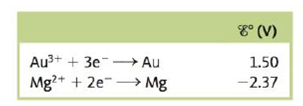Chapter 18, Problem 137CWP

Chapter
Section
Textbook Problem

# Consider a galvanic cell based on the following half-reactions:a. What is the standard potential for this cell?b. A nonstandard cell is set up at 25°C with [Mg2+] = 1.00 × 10−5 M. The cell potential is observed to be 4.01 V. Calculate [Au3+] in this cell.

(a)

Interpretation Introduction

Interpretation:

The half-reactions taking place in a galvanic cell is given. The value of standard cell potential and the concentration of Au3+ is to be calculated.

Concept introduction:

The relationship between reduction potential and standard reduction potential value and activities of species present in an electrochemical cell at a given temperature is given by the Nernst equation.

The value of Ecell is calculated using Nernst formula,

E=E°(RTnF)ln(Q)

At room temperature, the above equation specifies as,

E=E°(0.0591n)log(Q)

To determine: The value of the standard cell potential for the given cell.

The value of standard cell potential is 3.87V_ .

Explanation

The reaction taking place at the cathode is,

Au3++3eAuE°red=1.50V

The reaction taking place at the anode is,

MgMg2++2eE°OX=2.37V

Multiply reduction half-reaction with a coefficient of 2 and oxidation half-reaction with a coefficient of 3 and then add both the reactions as,

2Au3++6e2Au3Mg3Mg2++6e

The final equation is,

2Au3++3Mg2Au+3Mg2+ </

(b)

Interpretation Introduction

Interpretation:

The half-reactions taking place in a galvanic cell is given. The value of standard cell potential and the concentration of Au3+ is to be calculated.

Concept introduction:

The relationship between reduction potential and standard reduction potential value and activities of species present in an electrochemical cell at a given temperature is given by the Nernst equation.

The value of Ecell is calculated using Nernst formula,

E=E°(RTnF)ln(Q)

At room temperature, the above equation specifies as,

E=E°(0.0591n)log(Q)

To determine: The concentration of Au3+ for the given cell.

The concentration of Au3+ in the cell is 6.02×108M_ .

### Still sussing out bartleby?

Check out a sample textbook solution.

See a sample solution

#### The Solution to Your Study Problems

Bartleby provides explanations to thousands of textbook problems written by our experts, many with advanced degrees!

Get Started